# Frequency Modulation (FM)

## Overview

Frequency Modulation (FM) is a form of modulation in which changes in the carrier wave frequency correspond directly to changes in the baseband signal. FM is considered an analog form of modulation because the baseband signal is typically an analog waveform without discrete, digital values.

## Common Applications

Frequency modulation (FM) is most commonly used for radio and television broadcast. The FM band is divided between a variety of purposes. Analog television channels 0 through 72 utilize bandwidths between 54 MHz and 825 MHz. In addition, the FM band also includes FM radio, which operates from 88 MHz to 108 MHz. Each radio station utilizes a 38 kHz frequency band to broadcast audio.

## FM Theory

The basic principle behind FM is that the amplitude of an analog baseband signal can be represented by a slightly different frequency of the carrier. We represent this relationship in the graph below.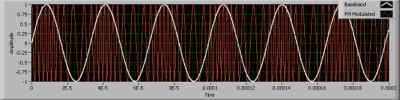Figure 1. Frequency Modulation

As this graph illustrates, various amplitudes of the baseband signal (shown in white) relate to specific frequencies of the carrier signal (shown in red). Mathematically, we represent this by describing the equations which characterize FM.

First, we represent our message, or baseband, signal by the simple designation m(t). Second, we represent a sinusoidal carrier by the equation:

xc(t) = Ac cos (2πfct).

The actual mathematical process to modulate a baseband signal, m(t), onto the carrier requires a two-step process. First, the message signal must be integrated with respect to time to get an equation for phase with respect to time, θ(t). This integration enables the modulation process because phase modulation is fairly straightforward with typical I/Q modulator circuitry. A block diagram description of an FM transmitter follows.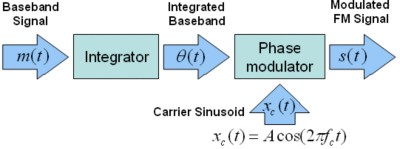Figure 2. FM Transmitter Block Diagram

As the block diagram above illustrates, the integration of a message signal results in an equation for phase with respect to time. This equation is defined by the following equation: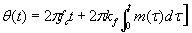where kf is the frequency sensitivity. Again, the resulting modulation that must occur is phase modulation, which involves changing the phase of the carrier over time. This process is fairly straightforward and requires a quadrature modulator, shown below.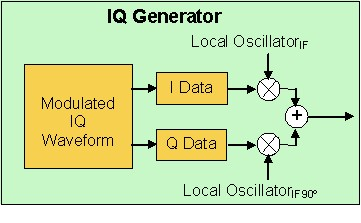Figure 3. Quadrature Modulator

As a result of phase modulation, the resulting FM signal, s(t), now represents the frequency modulated signal. This equation is shown below.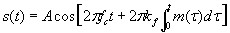where m(τ) = M cos (2πfmτ). More simply, we can also represent this equation as: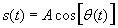## Modulation Index

One important aspect of frequency modulation is the modulation index. We already have established that changes in amplitude of the baseband correspond to changes in carrier frequency. The factor that determines exactly how much the carrier deviates from its center frequency is known as the modulation index. Mathematically, we have already identified our integrated baseband signal as the following equation.We can simplify this equation to the following: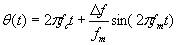In the equation above, ∆ƒ is the frequency deviation, which represents the maximum frequency difference between the instantaneous frequency and the carrier frequency. In fact, the ratio of ∆ƒ to the carrier frequency is the modulation index. This index, β, is thus defined by the equation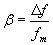The integrated message signal can be represented as: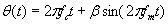As a result, we can substitute this new representation of θ(t) into our original formula to represent the final modulated FM signal as the following equation: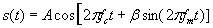The modulation index affects the modulated sinusoid in that the larger the modulation index, the greater the instantaneous frequency can be from the carrier. Below we illustrate an FM modulated signal in which the center frequency is 500 kHz. In the graph below, the FM deviation has been selected as 425 kHz. As a result, the modulated signal will have instantaneous frequencies from 75 kHz to 925 kHz. The wide range of frequencies is evident by observing the minimum amplitude of the baseband, when the modulated frequency is very small.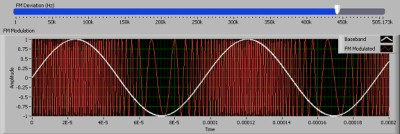Figure 4. FM Signal with 425 kHz FM Deviation

Contrast the image above to an FM signal where the frequency deviation is smaller. Below, we have chosen a 200 kHz FM deviation instead.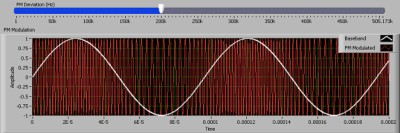Figure 5. FM Signal with 200 kHz FM Deviation

As Figure 5 illustrates, the instantaneous frequency range of the modulated signal is much smaller with a smaller FM deviation.

This property can be more fully observed by selecting the FM example.

## Conclusions

Frequency Modulation (FM) is an important modulation scheme both because of its widespread commercial use, and because of its simplicity. As we have seen in this document, frequency modulation can be simplified to angle modulation with a simple integrator. As a result, we can generate frequency modulated signals with the National Instruments vector signal generator, because they require nothing more than an I/Q modulator.

## Related Products

NI PXIe-5663 6.6 GHz RF Vector Signal Analyzer
The National Instruments PXIe-5663 is a modular 6.6 GHz RF vector signal analyzer with 50 MHz of instantaneous bandwidth optimized for automated test.

NI PXIe-5673 6.6 GHz RF Vector Signal Generator
The National Instruments PXIe-5673 is a 4-slot 6.6 GHz RF vector signal generator that delivers signal generation from 85 MHz to 6.6 GHz, 100 MHz of instantaneous bandwidth, and up to 512 MB of memory.

NI PXI-5660 2.7 GHz RF Vector Signal Analyzer
The National Instruments PXI-5660 is a modular 2.7 GHz RF vector signal analyzer with 20 MHz of instantaneous bandwidth optimized for automated test.

NI PXI-5671 2.7 GHz RF Vector Signal Generator
The National Instruments PXI-5671 module is a 3-slot RF vector signal generator that delivers signal generation from 250 kHz to 2.7 GHz, 20 MHz of instantaneous bandwidth, and up to 512 MB of memory.

NI PXI-5652 6.6 GHz RF and Microwave Signal Generator
The National Instruments PXI-5652 6.6 GHz RF and microwave signal generator is continuous-wave with modulation capability. It is excellent for setting up stimulus response applications with RF signal analyzers.

NI RF Switches
The National Instruments RF switch modules are ideal for expanding the channel count or increasing the flexibility of systems with signal bandwidths greater than 10 MHz to bandwidths as high as 26.5 GHz.

NI LabVIEW
National Instruments LabVIEW is an industry-leading graphical software tool for designing test, measurement, and automation systems.

NI LabWindows/CVI
National Instruments LabWindows/CVI is an a proven ANSI C integrated development environment that provides engineers and scientists with a comprehensive set of programming tools for creating test and control applications. LabWindows/CVI combines the longevity and reusability of ANSI C with engineering-specific functionality designed for instrument control, data acquisition, analysis, and user interface development.

## References

1. Haykin, Simon. Communications Systems. 3rd ed. Canada: John Wiley & Sons, Inc., 1994.
2. Lathi, B. P. Modern Digital and Analog Communication Systems. 3rd ed. USA: Oxford University Press, 1998.